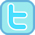﻿ Multiplication and Division
 HOME ABOUT US SHOP PRIVACY CONTACT# Multiplication and Division Worksheets and Resources

### Free multiplication and division worksheets, interactive activities, games and other resources for 5 to 11 year olds.

 Early Multiplication and Division Skills Doubles (to double 6) Doubles to Double 12 / Halves to Half of 24 Doubles to Double 20 / Halves to Half of 40 Doubles to Double 50 / Halves to Half of 100 1x Table / Division by 1 10x Table / Division by 10 2x Table / Division by 2 5x Table / Division by 5 Year 2 Times Tables and Division Facts 3x Table / Division by 3 4x Table / Division by 4 Year 3 Times Tables & Division Facts 6x Table / Division by 6 7x Table / Division by 7 8x Table / Division by 8 9x Table / Division by 9 1x to 10x Mixed Tables and Division Facts 11x Table / Divisiion by 11 12x Table / Division by 12 Times Table and Division Fact Practice Multiplication / Division Fact Families Multiples, Factors and Prime Numbers Place Value Multiplication & Division 2-digit Multiplied By 1-digit Written Multiplication & Division Strategies Other Multiplication & Division Resources